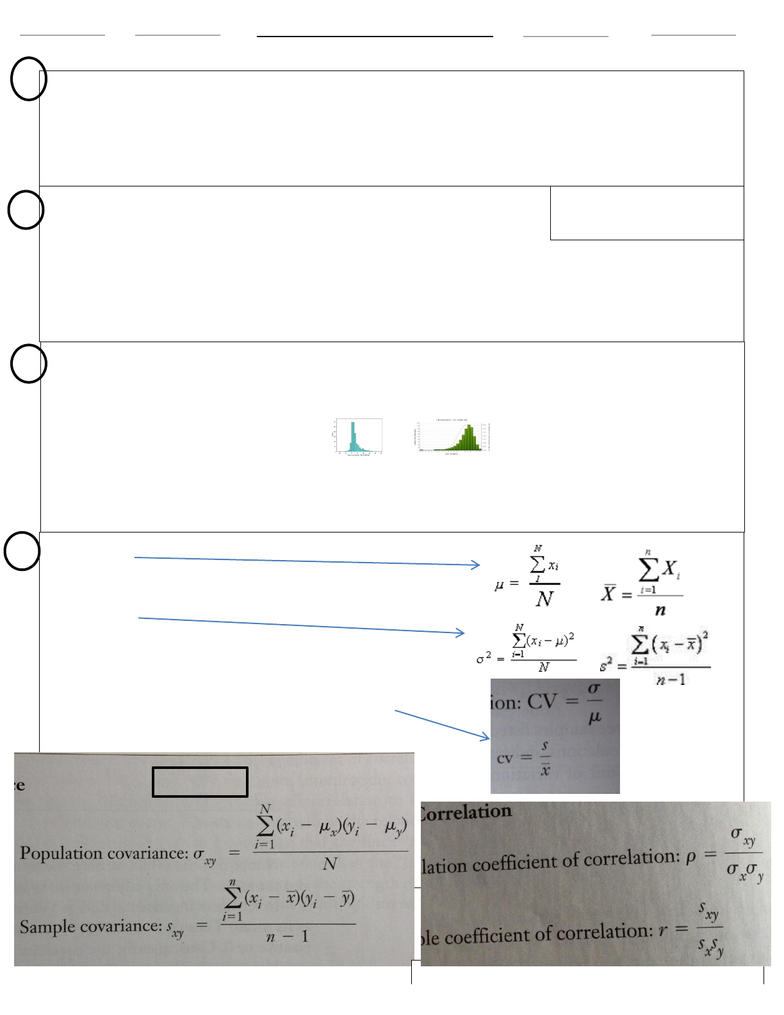Study Guides (390,000)
CA (150,000)
York (10,000)
Quiz

ADMS 2320 Study Guide - Quiz Guide: Conditional Probability, Type I And Type Ii Errors, Level Of Measurement

Department
Course Code
Professor
Marwan Hassan
Study Guide
Quiz

This preview shows half of the first page. to view the full 3 pages of the document.-Descriptive stats deals with organizing, summarizing, presenting data in a convenient/informative/useful way.
-Inferential stats is a body of methods used to draw conclusions or inferences about characteristics of population based on sample data
-Population is the group of all items of interest to a statistics practitioner(entire set of observations under study) descriptive measure is parameter
-Sample is a set of data drawn from the studied population(is a subset of a population) descriptive measure for samp is statistic
-Statistical inference is the process of making an estimate, prediction, decision about a population based on sample data
-Confidence level is the proportion of times that an estimating procedure will be correct
-Significance level measures how frequently the conclusion will be wrong
-Descriptive stats includes graphical and tabular statistical methods
-Variable is some characteristic of a population/sample
-Values of variable are the possible observations of the variable
-Data are the observed values of a variable
-Interval data are real numbers (height/weight/distance) Also called quantitive or numerical
-Nominal data are categories (1=married, 2=single) Also called qualitative or categorical
-Ordinal data show that the order of the values have meaning (poor fair good v.good)
- Two methods for nominal data are graphical/tabular freq.distribution/rel.freq.distribution bar chart/pie chart
- Techniques applied to single set of data is univirate; bivirate. to compare two nominal data used classification cross / bar chart(2&more)
Interval may be T into ordinal/nominal
Ordinal may be T into nominal
Nominal X
-Number of Classes 1+3.3log(n) n=no of observations
-Class width Range/Classes
-Three shapes of histograms 1. Symmetrical 2. Positively Skewed 3. Negatively Skewed
-Unimodal has one peak, Bimodal has two peaks.
-Bell-shaped histogram
-Ogive cumulative relative frequency on y axis
-For interval data use ogive
-For time-series data use line chart
- Relationship between two variables is measured by scatter diagram
-Three relationships 1.Strong linear relationship 2. Medium-strength linear “” 3. Weak linear “”
- Positive and negative linear relationship
-Non-linear relationship
-Measures of central location
-Mean
-Median place in order, select middle two or one
-Mode most common
-Range = Largest Smallest
-Variance
-Standard Deviation is sq.root of variance
-Chebysheffs Theorem the proportion of observations in any sam. That lie
- Within K standard deviations of the mean is at least 1-1/k2
-
-Coefficient of variation is the set of observations in the st.dev of the observations
- Divided by their mean pop coeff CV= o/u sample coeff cv = s/x
1
Formula Sheet ADMS 2320 Prof. Yogi
3
4
2
Formula Sheet
Formula Sheet
Formula Sheet
Formula Sheet
pop
pop
deviations of the variable
Covariance
You're Reading a Preview

Unlock to view full version